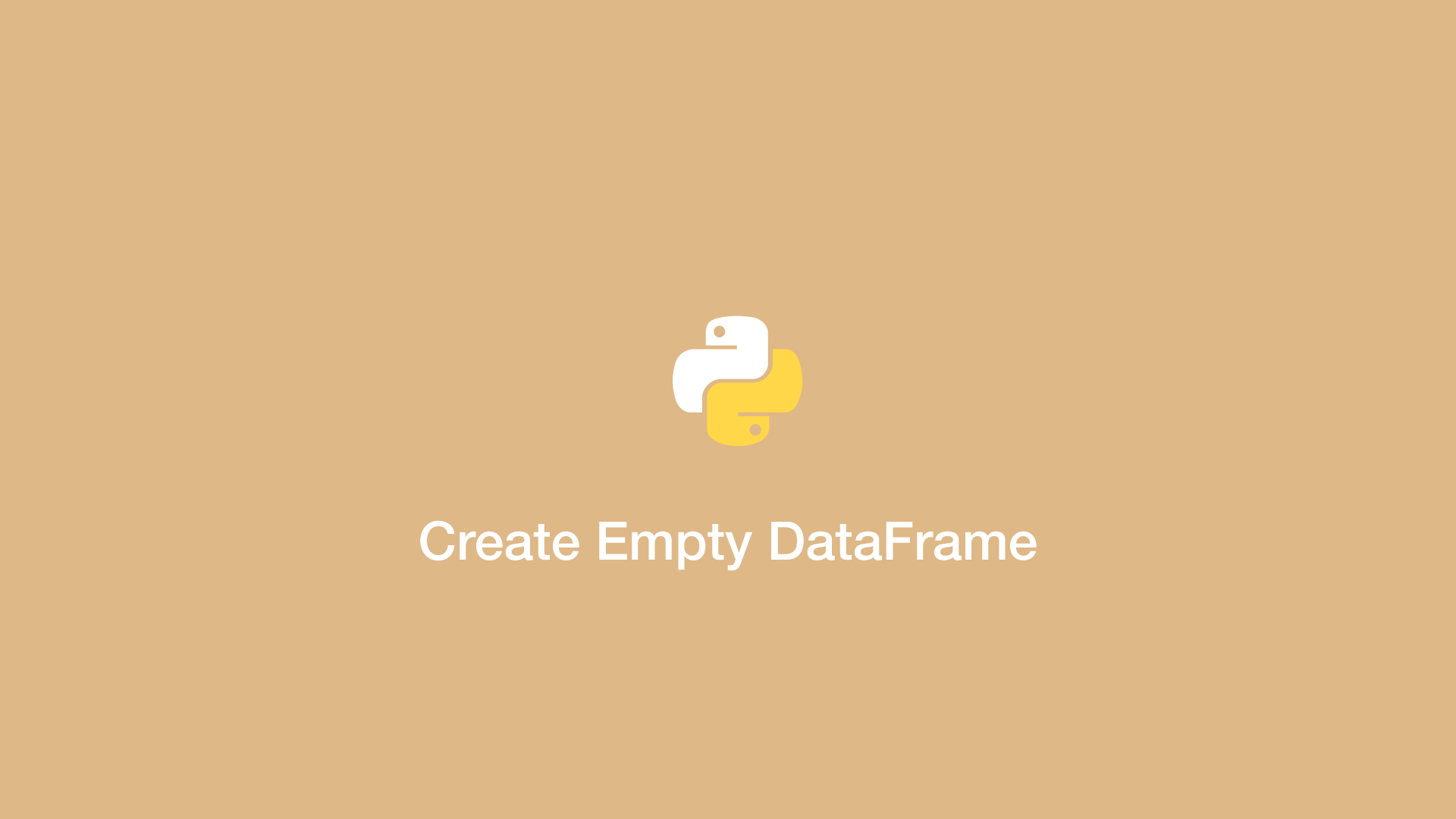# How to Create an Empty DataFrame with Column Names in PythonA DataFrame in Pandas is a table data structure containing rows and columns. In this tutorial, we will learn how to create an empty Pandas DataFrame with named columns and no values.

## Import Pandas

The first step is to ensure you have imported Pandas into your Python program before where you intend to create a DataFrame.

``````import pandas
``````

## Creating the DataFrame

Now let's create the DataFrame. This is done using the `pandas.DataFrame()` method and passing `columns =` followed by a `list` of column names as the first argument.

``````data_frame = pandas.DataFrame(columns = ['a', 'b', 'c'])

print(data_frame)
``````
``````Empty DataFrame
Columns: [a, b, c]
Index: []
``````

#### Related Tutorials### How to Replace NA or NaN Values in Pandas DataFrame with fillna()

June 10, 2021### How to Return a Column of a pandas DataFrame as a list in Python

April 21, 2021### How to Add or Insert Row to Pandas DataFrame

June 10, 2021June 22, 2020